【贝壳网】贝壳搜索为什么能知道你想住哪？1. 项目背景

2. 相关算法

3. 训练集的生成过程

4. 进行过的对比实验

5. 总结与展望

6. 项目背景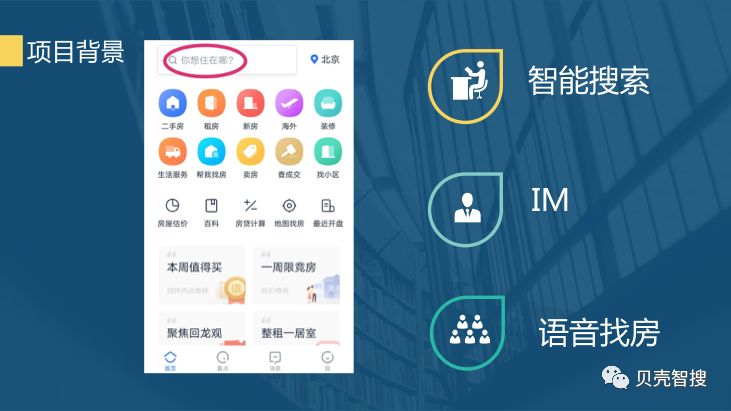1. 算法概览

BiLSTM-CRF 的混合方法，目前已成为实体识别的主流。BiLSTM 层负责每个字对应每个 label 的得分，CRF 层能够学习到数据中的序列约束，负责保证句子序列的正确性。整个模型的结构如下图所示: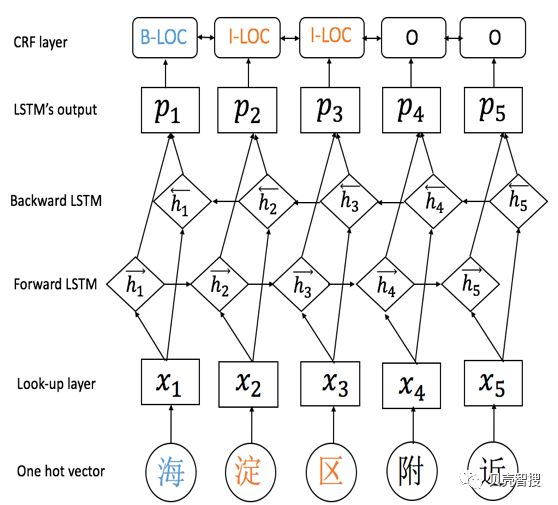• 第一层是 word-embedding 层。

• 第二层是 BiLSTM 层。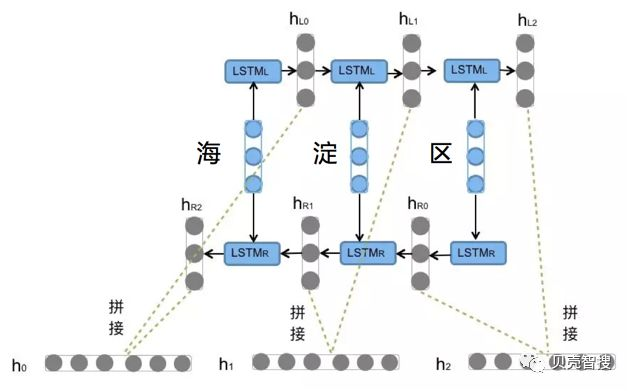• 第三层是 CRF 层。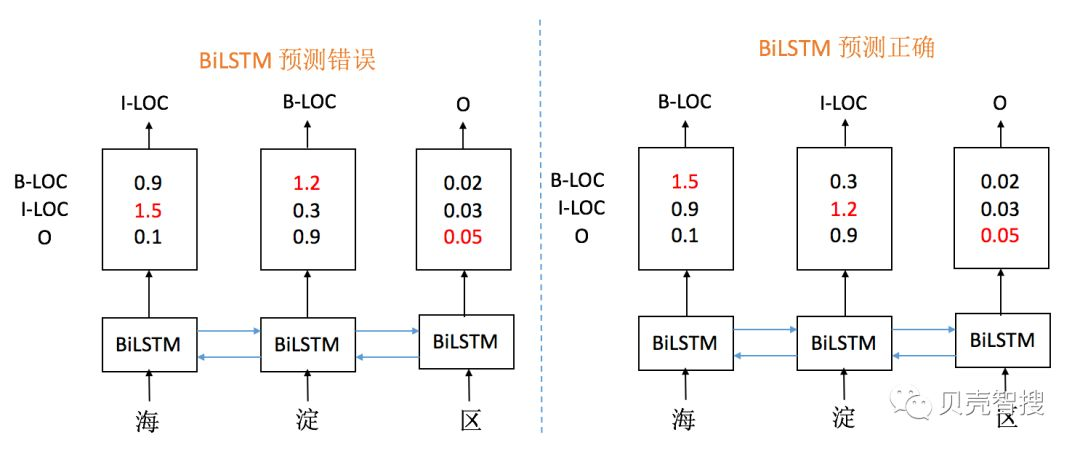2. 标签序列“O I-label” is 非法的。实体标签的首个标签应该是 “B-“ ，而非 “I-“, 换句话说, 有效的标签序列应该是“O B-label”。

CRF 层的参数是一个 K * K 的矩阵 A, Aij 表示的是从第 i 个标签到第 j 个标签的转移得分，进而在为一个位置进行标注的时候可以利用此前已经标注过的标签，如果记一个长度等于句子长度的标签序列 y=(y1,y2,…,yn)，那么模型对于句子 x 的标签等于 y 的打分为: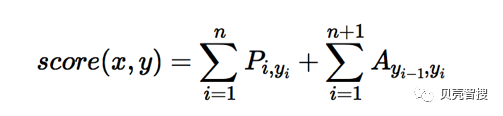1. 训练集的生成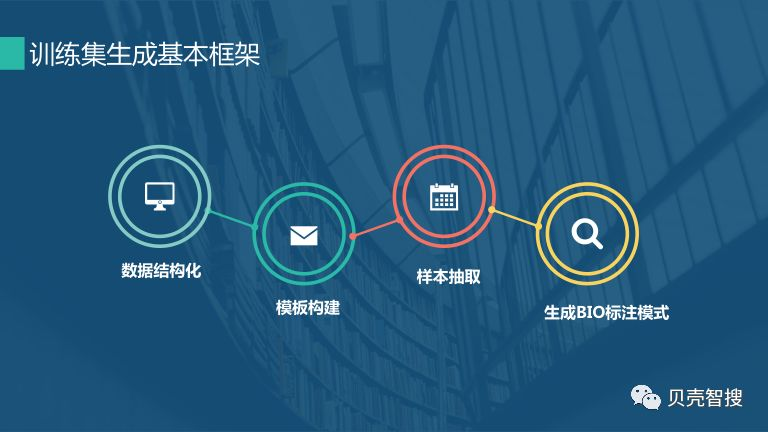3.2 训练集的构造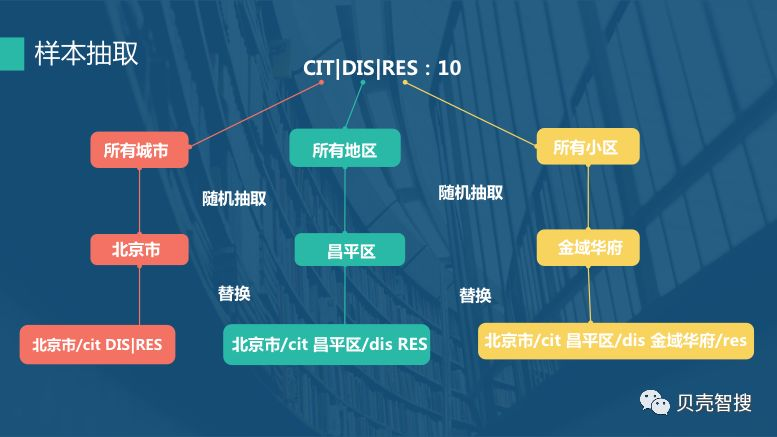1.  `如下所示为 DET--房屋单元  的部分模板与设置的权重`

2.  `房屋单元:(带|有|包括|)(露台|阳台|平台|落地窗|飘窗)  2`

3.  `房屋单元:带(花园|院|阁楼)  3`

4.  `房屋单元:(双天井|双阳台|大阳台|大露台|大平台|大落地窗)  1`

5.  `房屋单元:x(居|室|房|厅)  1`

6.  `房屋单元:x(房|室)x厅  5`

7.  `房屋单元:x(室|房)x厅(1|一)厨  1`

8.  `房屋单元:x(室|房)x厅(1|2|一|二)卫  2`

9.  `房屋单元:x(室|房)x厅(1|一)厨(1|2|一|二)卫  1`

10.  `房屋单元:x(室|房)x厅(1|2|一|二)卫(1|一)厨  1`

11.  `房屋单元:x(居|居室)  10`

12.  `房屋单元:x(居|居室)(到|至|-|~|或者|或|)x(居|居室)  5`

1. 对比实验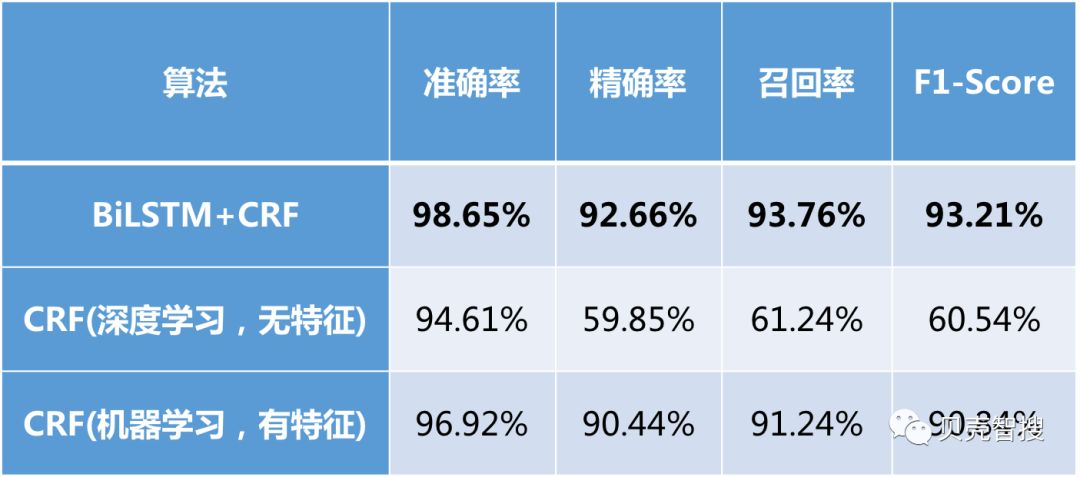1.  `# BiLSTM层`

2.  `def biLSTM_layer_op(self):`

3.   `with tf.variable_scope("bi-lstm"):`

4.   `# 计算前向后向参数`

5.   `cell_fw =  LSTMCell(self.hidden_dim)`

6.   `cell_bw =  LSTMCell(self.hidden_dim)`

7.   `(output_fw_seq,output_bw_seq), _ =`

8.   `tf.nn.bidirectional_dynamic_rnn(`

9.   `cell_fw=cell_fw,`

10.   `cell_bw=cell_bw,`

11.   `inputs=self.word_embeddings,`

12.   `sequence_length=self.sequence_lengths,`

13.   `dtype=tf.float32)`

14.   `# 参数全连接`

15.   `output = tf.concat([output_fw_seq,output_bw_seq], axis=-1)`

16.   `output = tf.nn.dropout(output, self.dropout_pl)`

17.   `# 通过一个线性层,得到每个标签的得分`

18.   `# 当只有CRF模型时，只有该线性层，不经过上述参数计算且维度不需乘2`

19.   `with tf.variable_scope("proj"):`

20.   `W = tf.get_variable(`

21.   `name="W",`

22.   `shape=[2  * self.hidden_dim,self.num_tags],`

23.   `initializer=tf.contrib.layers.xavier_initializer(),`

24.   `dtype=tf.float32)`

25.

26.   `b = tf.get_variable(`

27.   `name="b",`

28.   `shape=[self.num_tags],`

29.   `initializer=tf.zeros_initializer(),`

30.   `dtype=tf.float32)`

31.

32.   `s = tf.shape(output)`

33.   `output = tf.reshape(output,  [-1,  2  * self.hidden_dim])`

34.   `pred = tf.add(tf.matmul(output, W), b, name='pred')`

35.   `self.logits = tf.reshape(`

36.   `pred,  [-1, s, self.num_tags], name='logits')`

37.

38.

39.  `#CRF层`

40.  `def CRF(self):`

41.   `#得到转移矩阵`

42.   `log_likelihood, self.transition_params = crf_log_likelihood(`

43.   `inputs=self.logits,`

44.   `tag_indices=self.labels,`

45.   `sequence_lengths=self.sequence_lengths)`

46.   `self.loss =  -tf.reduce_mean(log_likelihood)`

47.   `#解码`

48.   `self.outputs, _ = crf_decode(`

49.   `potentials=self.logits,`

50.   `transition_params=self.transition_params,`

51.   `sequence_length=self.sequence_lengths)`

52.   `self.outputs0 = tf.reshape(`

53.   `self.outputs,  [-1, self.sequence_lengths], name='outputs')`

1. 总结与展望

参考文献

 Zhiheng Huang,Wei Xu,Kai Yu.Bidirectional LSTM-CRF Models for Sequence Tagging.arXiv:1508.01991v1 [cs.CL] 9 Aug 2015

 Xuezhe Ma and Eduard Hovy.End-to-end Sequence Labeling via Bi-directional LSTM-CNNs-CRF